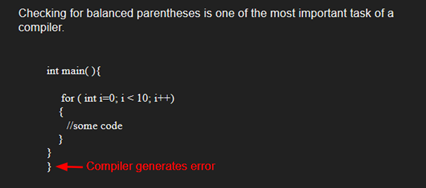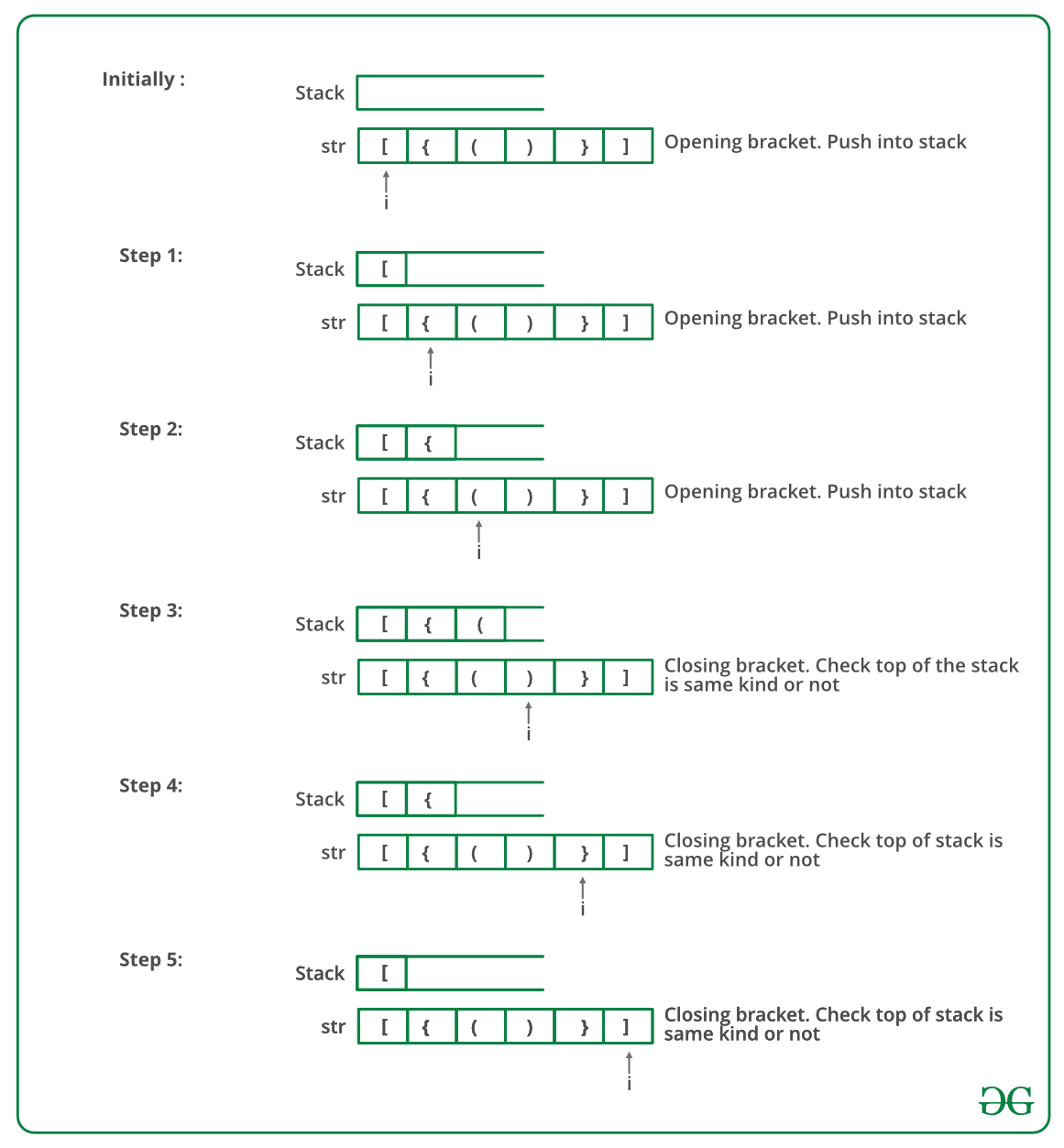# Python Program To Check For Balanced Brackets In An Expression (Well-Formedness) Using Stack

Given an expression string exp, write a program to examine whether the pairs and the orders of “{“, “}”, “(“, “)”, “[“, “]” are correct in exp.

Example

Input: exp = “[()]{}{[()()]()}”
Output: Balanced

Input: exp = “[(])”
Output: Not BalancedAlgorithm:

• Declare a character stack S.
• Now traverse the expression string exp.
1. If the current character is a starting bracket (‘(‘ or ‘{‘ or ‘[‘) then push it to stack.
2. If the current character is a closing bracket (‘)’ or ‘}’ or ‘]’) then pop from stack and if the popped character is the matching starting bracket then fine else brackets are not balanced.
• After complete traversal, if there is some starting bracket left in stack then “not balanced”

Below image is a dry run of the above approach:Below is the implementation of the above approach:

## Python3

 `# Python3 program to check for ` `# balanced brackets. ` ` `  `# function to check if ` `# brackets are balanced ` ` `  ` `  `def` `areBracketsBalanced(expr): ` `    ``stack ``=` `[] ` ` `  `    ``# Traversing the Expression ` `    ``for` `char ``in` `expr: ` `        ``if` `char ``in` `[``"("``, ``"{"``, ``"["``]: ` ` `  `            ``# Push the element in the stack ` `            ``stack.append(char) ` `        ``else``: ` ` `  `            ``# IF current character is not opening ` `            ``# bracket, then it must be closing. ` `            ``# So stack cannot be empty at this point. ` `            ``if` `not` `stack: ` `                ``return` `False` `            ``current_char ``=` `stack.pop() ` `            ``if` `current_char ``=``=` `'('``: ` `                ``if` `char !``=` `")"``: ` `                    ``return` `False` `            ``if` `current_char ``=``=` `'{'``: ` `                ``if` `char !``=` `"}"``: ` `                    ``return` `False` `            ``if` `current_char ``=``=` `'['``: ` `                ``if` `char !``=` `"]"``: ` `                    ``return` `False` ` `  `    ``# Check Empty Stack ` `    ``if` `stack: ` `        ``return` `False` `    ``return` `True` ` `  ` `  `# Driver Code ` `if` `__name__ ``=``=` `"__main__"``: ` `    ``expr ``=` `"{()}[]"` ` `  `    ``# Function call ` `    ``if` `areBracketsBalanced(expr): ` `        ``print``(``"Balanced"``) ` `    ``else``: ` `        ``print``(``"Not Balanced"``) ` ` `  `# This code is contributed by AnkitRai01 and improved ` `# by Raju Pitta `

Output

`Balanced`

Time Complexity: O(n)
Auxiliary Space: O(n) for stack.

Please refer complete article on Check for Balanced Brackets in an expression (well-formedness) using Stack for more details!

Feeling lost in the world of random DSA topics, wasting time without progress? It's time for a change! Join our DSA course, where we'll guide you on an exciting journey to master DSA efficiently and on schedule.
Ready to dive in? Explore our Free Demo Content and join our DSA course, trusted by over 100,000 geeks!

Previous
Next